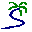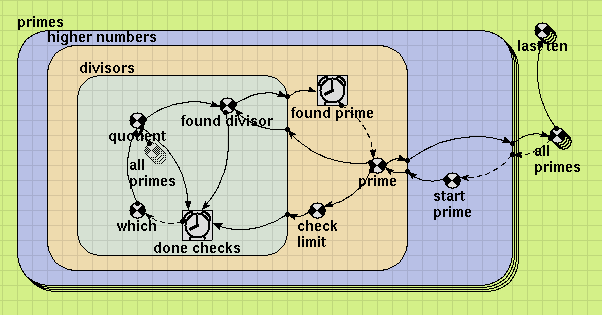# Model entry

Generating prime numbers: graphical programming with Simile - Model catalogue - Simulistics.com

 Search Simulistics Model catalogue Listed by keyword Listed by ID Listed by title Listed by date added

# Generating prime numbers: graphical programming with Simile

Model : Primes
Simile version : 4.0
Keywords : Iteration ; Alarm ;

## Description

This model generates an array containing the first 500,000 prime numbers. I built it in order to:
• Demonstrate the use of the iterative submodel feature, new to Simile 4.0
• Show that Simile models can be used instead of programs in text-based procedural languages such as FORTRAN for many problems, and are more comprehensible and easier to learn as a technique than programs in such languages.

An alarm symbol is given a boolean value. If a submodel contains an alarm symbol, then each time step its contents will be evaluated once in any case, then repeatedly until the alarm symbol has the value TRUE. If alarm submodels are nested, the inner one goes through the process of repeatedly evaluating each time the outer one evaluates. This is analogous to nested loops in procedural languages.

Also new to 4.0 is the dashed influence arrow. This replaces the 'sofar()' function in some earlier versions of Simile, and it means that evaluation of the function at its end is not to wait for the value at its start. The value used can therefore be from a previous iteration, or the previous index in an array, or even the last time step. Using dashed arrows allows models to contain circular chains of influences. When using this arrow (or prev(0), which means take the previous value of the function you are calculating) you need to be careful to include a boundary condition so it is not used when there is no previous value. e.g., if time()>0 then f(prev(0)) else 5.

In this model, we want to keep all the prime numbers that are found, so the outermost submodel has 500,000 instances. The array variable 'all primes' contains the results. 'start prime' is the value at which to start checking for prime numbers in each submodel instance. For the first instance it is 5; we cannot start with 3 as there are no divisors to check, since we will be skipping even numbers anyway. For subsequent instances it will be whatever prime number was found in the last one, plus two.

The submodel 'higher numbers' is iterative. When looking at this submodel, think of 'divisors' as a black box that simply indicates prime or composite for each number fed into it. 'higher numbers' uses it to check each number for being prime, starting with 'start prime', and adding two each time a number turns out to be composite. When the iteration ends, 'prime' is the first prime number greater than or equal to 'start prime'. This submodel also sets 'check limit' to the square root of each value being tested, so the submodel that actually does the testing knows where to stop.

The submodel 'divisors' is also iterative. It starts with the value 'which' which indicates which member of the array of primes so far discovered to try to divide the current candidate with next. This is set to 0 at the start of the iteration, and incremented by 1 each time it goes round. 'quotient' is the corresponding value picked from the array (a ghost variable is used to tidy the diagram), or 3 if 'which' is 0, since 3 does not appear in the results array. 'found divisor' is true if it divides exactly, and 'done checks' finishes the loop either if this is true (number not prime) or if the next quotient is larger than the square root (number prime).

The model is very efficient, since it only checks each prime candidate for divisibility by other primes up to its square root. It will generate 500,000 primes in a matter of seconds on a typical PC. It does all the calculation on initialization as it has no time-dependent values. However Simile's display tools are not up to displaying that many values, so the variable 'last ten' has been added to allow only the final 10 primes to be displayed.

## Files

### Model file

Click on the icon to download the model file. (You will need Simile to examine and run the model. A free evaluation version is available from the products page.)

Some browsers may attempt to display the model file, rather than open it in Simile; in this case, use the browser back button to return to this page, and use the context menu (invoked by right-clicking on the link) to save the target file to disk.primes.sml

## Diagram## Results

|Here is the popup window that appears (after a short delay) for the array variable containing all primes:|